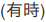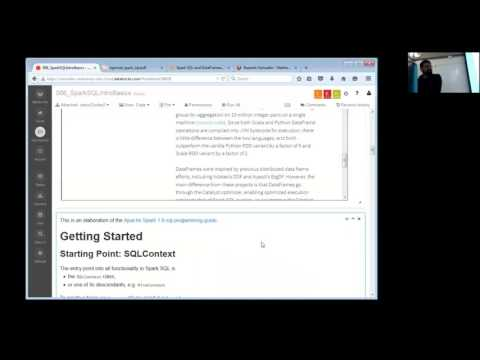// Databricks notebook source exported at Sat, 18 Jun 2016 07:46:37 UTC

# Scalable Data Science

### prepared by Raazesh Sainudiin and Sivanand Sivaram

The html source url of this databricks notebook and its recorded Uji:#Introduction to Spark SQL

• This notebook explains the motivation behind Spark SQL
• It introduces interactive SparkSQL queries and visualizations
• This notebook uses content from Databricks SparkSQL notebook and SparkSQL programming guide

Some resources on SQL

Some of them are embedded below in-place for your convenience.

``````
//This allows easy embedding of publicly available information into any other notebook
//when viewing in git-book just ignore this block - you may have to manually chase the URL in frameIt("URL").
//Example usage:
// displayHTML(frameIt("https://en.wikipedia.org/wiki/Latent_Dirichlet_allocation#Topics_in_LDA",250))
def frameIt( u:String, h:Int ) : String = {
"""<iframe
src=""""+ u+""""
width="95%" height="""" + h + """"
sandbox>
<p>
<a href="http://spark.apache.org/docs/latest/index.html">
Fallback link for browsers that, unlikely, don't support frames
</a>
</p>
</iframe>"""
}
displayHTML(frameIt("https://en.wikipedia.org/wiki/SQL",500))

``````
``````
displayHTML(frameIt("https://en.wikipedia.org/wiki/Apache_Hive#HiveQL",175))

``````
``````
displayHTML(frameIt("https://databricks.com/blog/2015/02/17/introducing-dataframes-in-spark-for-large-scale-data-science.html",600))

``````

This is an elaboration of the Apache Spark 1.6 sql-progamming-guide.

# Getting Started

## Starting Point: SQLContext

The entry point into all functionality in Spark SQL is

To create a basic `SQLContext`, all you need is a `SparkContext`.

Conveniently, in Databricks notebook (similar to `spark-shell`) `SQLContext` is already created for you and is available as `sqlContext`.

``````
// Cntrl+Enter will print sqlContext available in notebook
sqlContext

``````

Deeper Dive: (beginners skip for now)

Usually, when building application (running on production-like on-premises cluster) you would manually create `SQLContext` using something like this:

``````// An existing SparkContext
val sc: SparkContext = ...
val sqlContext = new org.apache.spark.sql.SQLContext(sc)

// This is used to implicitly convert an RDD to a DataFrame (see examples below)
import sqlContext.implicits._
``````

Note that SQLContext in notebook is actually HiveContext. The difference is that HiveContext provides richer functionality over standard SQLContext, e.g. window functions were only available with HiveContext up to Spark 1.5.2, or usage of Hive user-defined functions. This originates from the fact that Spark SQL parser was built based on HiveQL parser, so only HiveContext was supporting full HiveQL syntax. Now it is changing, and SQLContext supports most of the functionality of the descendant (window functions should be available in SQLContext in 1.6+).

Note that you do not need Hive installation when working with SQLContext or HiveContext, Spark comes with built-in derby datastore.

## Creating DataFrames

With a `SQLContext`, applications can create `DataFrame`

• from an existing `RDD`,
• from a Hive table, or
• from various other data sources.

#### Just to recap:

• A DataFrame is a distributed collection of data organized into named columns.
• You can think of it as being organized into table RDD of case class `Row` (which is not exactly true).
• DataFrames, in comparison to RDDs, are backed by rich optimizations, including:
• tracking their own schema,
• code generation including whole stage codegen,
• extensible Catalyst optimizer, and
• project Tungsten.

Note that performance for DataFrames is the same across languages Scala, Java, Python, and R. This is due to the fact that the only planning phase is language-specific (logical + physical SQL plan), not the actual execution of the SQL plan.## DataFrame Basics

#### 1. Making an empty DataFrame

Spark has some of the pre-built methods to create simple DataFrames

• let us make an Empty DataFrame
``````
val emptyDF = sqlContext.emptyDataFrame // Ctrl+Enter to make an empty DataFrame

``````

Not really interesting, or is it?

You Try!

Put your cursor after `emptyDF.` below and hit Tab to see what can be done with `emptyDF`.

``````
emptyDF.

``````

#### 2. Making a DataFrame from a range

Let us make a DataFrame next

• from a range of numbers, as follows:
``````
val rangeDF = sqlContext.range(0, 3) // Ctrl+Enter to make DataFrame with 0,1,2

``````

Note that Spark automatically names column as `id` and casts integers to type `bigint` for big integer or Long.

In order to get a preview of data in DataFrame use `show()` as follows:

``````
rangeDF.show() // Ctrl+Enter

``````

#### 3. Making a DataFrame from an RDD

• Make an RDD
• Conver the RDD into a DataFrame using the defualt `.toDF()` method
• Conver the RDD into a DataFrame using the non-default `.toDF(...)` method
• Do it all in one line

Let’s first make an RDD using the `sc.parallelize` method, transform it by a `map` and perform the `collect` action to display it, as follows:

``````
val rdd1 = sc.parallelize(1 to 5).map(i => (i, i*2))
rdd1.collect() // Ctrl+Enter

``````

Next, let us convert the RDD into DataFrame using the `.toDF()` method, as follows:

``````
val df1 = rdd1.toDF() // Ctrl+Enter

``````

As it is clear, the DataFrame has columns named `_1` and `_2`, each of type `int`. Let us see its content using the `.show()` method next.

``````
df1.show() // Ctrl+Enter

``````

Note that by default, i.e. without specifying any options as in `toDF()`, the column names are given by `_1` and `_2`.

We can easily specify column names as follows:

``````
val df1 = rdd1.toDF("single", "double") // Ctrl+Enter
df1.show()

``````

Of course, we can do all of the above steps to make the DataFrame `df1` in one line and then show it, as follows:

``````
val df1 = sc.parallelize(1 to 5).map(i => (i, i*2)).toDF("single", "double") //Ctrl+enter
df1.show()

``````

You Try!

Fill in the `???` below to get a DataFrame `df2` whose first two columns are the same as `df1` and whose third column named triple has values that are three times the values in the first column.

``````
val df2 = sc.parallelize(1 to 5).map(i => (i, i*2, ???)).toDF("single", "double", "triple") // Ctrl+enter after editing ???
df2.show()

``````

#### 4. Making a DataFrame from a table

We can load existing tables as DataFrames. We will later see how to create tables from RDDs or other sources of raw data, including csv files, etc.

First let’s see what tables are available to us.

``````
sqlContext.tables.show() // Ctrl+Enter to see available tables

``````

Let us load the table with `tableName` `diamonds` as a DataFrame (assuming it exixts!, if not don’t worry as diamonds is our next stop!), as follows:

``````
val diamondsDF = sqlContext.table("diamonds") // Shift+Enter

``````
``````
display(diamondsDF)

``````

### 5. Using SQL for interactively querying a table is very powerful!

Note `-- comments` are how you add `comments` in SQL cells beginning with `%sql`.

• You can run SQL `select *` statement to see all columns of the table, as follows:
• This is equivalent to the above `display(diamondsDF)’ with the DataFrame
``````
%sql
-- Ctrl+Enter to select all columns of the table
select * from diamonds

``````

## Next we will play with data

The data here is semi-structured tabular data (Tab-delimited text file in dbfs). Let us see what Anthony Joseph in BerkeleyX/CS100.1x had to say about such data.

### Key Data Management Concepts: Semi-Structured Tabular Data

(watch now 1:26):This week’s recommended homework and extra-work linked below will help further your understanding beyond the three example notebooks we will see next at:

This week’s recommended homework is a deep dive into the SparkSQL programming guide via a “databricksified” set of scala notebooks at:

Those who want to understand SparkSQL functionalities in more detail can see:

• video lectures in Module 3 of Anthony Joseph’s Introduction to Big Data edX course from the Community Edition (CE) of databricks
• NOTE on June 18 2016: AJ’s 2015 course is now already in databricks CE so won’t be re-fielded here in html/git-booked md, except in the .dbc archive of this 2016 instance of the scalable-data-science course - remarked (you should really see the 2016 version of Anthony Joseph + Ameet Talwarkar + Jon Bates edX course now… and Spark 2.0 will be another story I am sure…).

Anthony Joseph’s Introduction to Big Data edX course (in python using pySpark) has been added to this databricks shard at:

Updated: